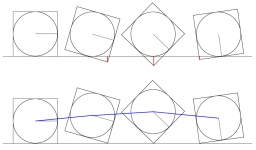# The schoolyard

The schoolyard had the shape of a square with an 11m side. The yard has been enlarged by 75 m2 and has a square shape again. How many meters was each side of the yard enlarged?

Result

x =  3 m

#### Solution:

$a = 11 \ m \ \\ S_{ 1 } = a^2 = 11^2 = 121 \ m^2 \ \\ S_{ 2 } = S_{ 1 } + 75 = 121 + 75 = 196 \ m^2 \ \\ \ \\ b = \sqrt{ S_{ 2 } } = \sqrt{ 196 } = 14 \ m \ \\ \ \\ x = b-a = 14-11 = 3 = 3 \ \text{ m }$Our examples were largely sent or created by pupils and students themselves. Therefore, we would be pleased if you could send us any errors you found, spelling mistakes, or rephasing the example. Thank you!

Leave us a comment of this math problem and its solution (i.e. if it is still somewhat unclear...):Be the first to comment!## Next similar math problems:

1. Annular areaThe square with side a = 1 is inscribed and circumscribed by circles. Find the annular area.
2. Company logoThe company logo consists of a blue circle with a radius of 4 cm, which is an inscribed white square. What is the area of the blue part of the logo?
3. Squares above sidesTwo squares are constructed on two sides of the ABC triangle. The square area above the BC side is 25 cm2. The height vc to the side AB is 3 cm long. The heel P of height vc divides the AB side in a 2: 1 ratio. The AC side is longer than the BC side. Calc
4. The sides 2The sides of a trapezoid are in the ratio 2:5:8:5. The trapezoid’s area is 245. Find the height and the perimeter of the trapezoid.
5. The trapeziumThe trapezium is formed by cutting the top of the right-angled isosceles triangle. The base of the trapezium is 10 cm and the top is 5 cm. Find the area of trapezium.
6. RectangleIn rectangle with sides, 6 and 3 mark the diagonal. What is the probability that a randomly selected point within the rectangle is closer to the diagonal than to any side of the rectangle?
7. Eq triangle minus arcsIn an equilateral triangle with a 2cm side, the arcs of three circles are drawn from the centers at the vertices and radii 1cm. Calculate the content of the shaded part - a formation that makes up the difference between the triangle area and circular cuts
8. Ratio of sidesCalculate the area of a circle that has the same circumference as the circumference of the rectangle inscribed with a circle with a radius of r 9 cm so that its sides are in ratio 2 to 7.
9. Area of a rectangleCalculate the area of a rectangle with a diagonal of u = 12.5cm and a width of b = 3.5cm. Use the Pythagorean theorem.
10. Trapezoid MOThe rectangular trapezoid ABCD with right angle at point B, |AC| = 12, |CD| = 8, diagonals are perpendicular to each other. Calculate the perimeter and area of ​​the trapezoid.
11. RectangleThere is a rectangle with a length of 12 cm and a diagonal 8 cm longer than the width. Calculate the area of rectangle.
12. 30-gonAt a regular 30-gon the radius of the inscribed circle is 15cm. Find the "a" side size, circle radius "R", circumference, and content area.
13. Trapezoid MO-5-Z8ABCD is a trapezoid that lime segment CE divided into a triangle and parallelogram as shown. Point F is the midpoint of CE, DF line passes through the center of the segment BE and the area of the triangle CDE is 3 cm2. Determine the area of the trapezoid
14. Circular railwayThe railway is to interconnect in a circular arc the points A, B, and C, whose distances are | AB | = 30 km, AC = 95 km, BC | = 70 km. How long will the track from A to C?
15. Rectangular fieldA rectangular field has a diagonal of length 169m. If the length and width are in the ratio 12:5. Find the dimensions of the field, the perimeter of the field and the area of the field.
16. Quarter circleWhat is the radius of a circle inscribed in the quarter circle with a radius of 100 cm?Show that the quadrilateral with vertices P1(0,1), P2(4,2) P3(3,6) P4(-5,4) has two right triangles.